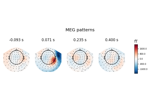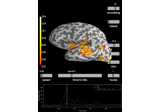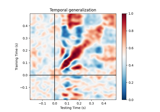# mne.decoding.LinearModel¶

class mne.decoding.LinearModel(model=None)[source]

Compute and store patterns from linear models.

The linear model coefficients (filters) are used to extract discriminant neural sources from the measured data. This class computes the corresponding patterns of these linear filters to make them more interpretable 1.

Parameters
modelobject | None

A linear model from scikit-learn with a fit method that updates a coef_ attribute. If None the model will be LogisticRegression.

Notes

New in version 0.10.

References

1

Stefan Haufe, Frank Meinecke, Kai Görgen, Sven Dähne, John-Dylan Haynes, Benjamin Blankertz, and Felix Bießmann. On the interpretation of weight vectors of linear models in multivariate neuroimaging. NeuroImage, 87:96–110, 2014. doi:10.1016/j.neuroimage.2013.10.067.

Attributes
filters_ndarray, shape ([n_targets], n_features)

If fit, the filters used to decompose the data.

patterns_ndarray, shape ([n_targets], n_features)

If fit, the patterns used to restore M/EEG signals.

Methods

 __hash__(/) Return hash(self). Compute distance from the decision function of y from X. fit(X, y, **fit_params) Estimate the coefficients of the linear model. fit_transform(X, y) Fit the data and transform it using the linear model. get_params([deep]) Get parameters for this estimator. Compute predictions of y from X. Compute probabilistic predictions of y from X. score(X, y) Score the linear model computed on the given test data. set_params(**params) Set the parameters of this estimator. Transform the data using the linear model.
decision_function(X)[source]

Compute distance from the decision function of y from X.

Parameters
Xarray, shape (n_samples, n_features)

The data used to compute the predictions.

Returns
y_predarray, shape (n_samples, n_classes)

The distances.

fit(X, y, **fit_params)[source]

Estimate the coefficients of the linear model.

Save the coefficients in the attribute filters_ and computes the attribute patterns_.

Parameters
Xarray, shape (n_samples, n_features)

The training input samples to estimate the linear coefficients.

yarray, shape (n_samples, [n_targets])

The target values.

**fit_paramsdict of str -> object

Parameters to pass to the fit method of the estimator.

Returns
selfinstance of LinearModel

Returns the modified instance.

Examples using fit:fit_transform(X, y)[source]

Fit the data and transform it using the linear model.

Parameters
Xarray, shape (n_samples, n_features)

The training input samples to estimate the linear coefficients.

yarray, shape (n_samples,)

The target values.

Returns
y_predarray, shape (n_samples,)

The predicted targets.

get_params(deep=True)[source]

Get parameters for this estimator.

Parameters
deepbool, optional

If True, will return the parameters for this estimator and contained subobjects that are estimators.

Returns
paramsmapping of str to any

Parameter names mapped to their values.

predict(X)[source]

Compute predictions of y from X.

Parameters
Xarray, shape (n_samples, n_features)

The data used to compute the predictions.

Returns
y_predarray, shape (n_samples,)

The predictions.

predict_proba(X)[source]

Compute probabilistic predictions of y from X.

Parameters
Xarray, shape (n_samples, n_features)

The data used to compute the predictions.

Returns
y_predarray, shape (n_samples, n_classes)

The probabilities.

score(X, y)[source]

Score the linear model computed on the given test data.

Parameters
Xarray, shape (n_samples, n_features)

The data to transform.

yarray, shape (n_samples,)

The target values.

Returns
scorefloat

Score of the linear model.

set_params(**params)[source]

Set the parameters of this estimator. The method works on simple estimators as well as on nested objects (such as pipelines). The latter have parameters of the form <component>__<parameter> so that it’s possible to update each component of a nested object. Returns ——- self

transform(X)[source]

Transform the data using the linear model.

Parameters
Xarray, shape (n_samples, n_features)

The data to transform.

Returns
y_predarray, shape (n_samples,)

The predicted targets.

## Examples using mne.decoding.LinearModel¶